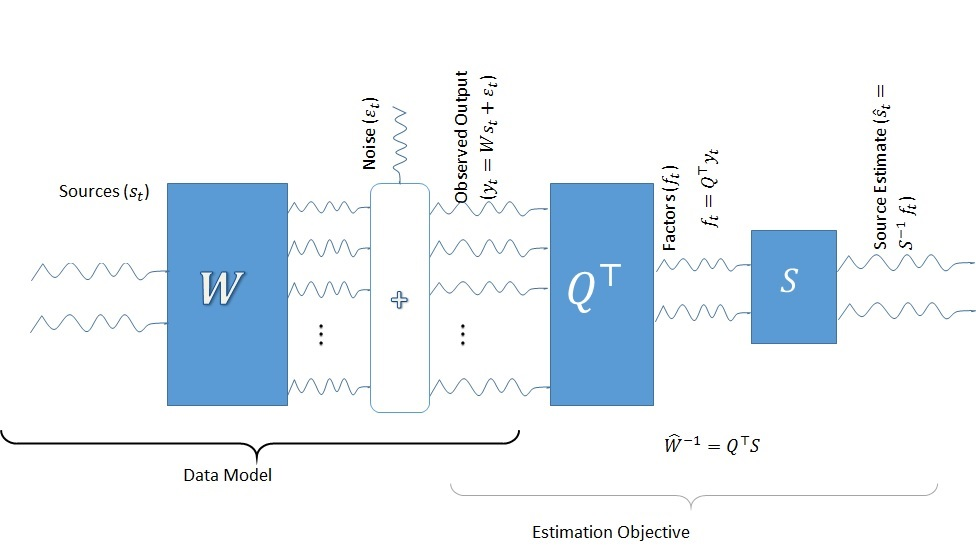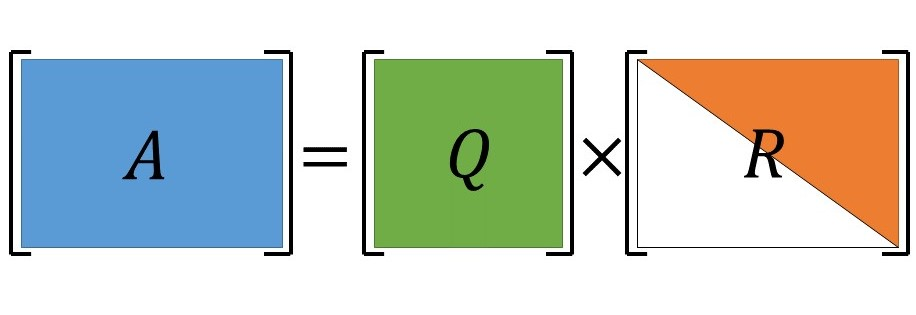# A view of the person and profession

## Main Content

### Teaching

Understanding is sharpened with teaching.

My favorite courses to teach are related to the fields of  Signal Processing, Statistics, Linear Algebra, Data Analysis, Convex Optimization and Communication Networks. This page lists all the courses taught and information and materials related to the course.

1. ECE 370 - Signals and Systems (For an example Lecture, click here, More detials and course materials, click here#### Signals and Systems

A project based approach to signals and systems covering all the traditional topics like correlation, convolution, Fourier Analysis, Laplace and Z- Transforms

each student will be required to complete a project involving the concepts taught in class to cement their understanding. The projects range from Using Software Defined Radio, Simulation of AM, FM, Understanding Wavelet Transform as correlation and using it with music signals, ECG signal processing, Understanding Echoes, ...

More details here.#### Linear Algebra

Students learn the concepts of linear algebra through practical problems and design solutions. Helps them think in terms of matrices and understand the properties like vector spaces and working of Fast Fourier Transform (FFT).

Book: Matrix Analysis: A practical Approach by C.D. Meyer

Software used: MATLAB to demonstrate and visualize applications that stem from Linear Algebra and veify the various properties and theorems.#### Communication Systems

Introduces mathematical concepts to understand communication systems (eg: modulation and demodulation), Understand the working and implementation of AM, FM modeling.

(lab): Build a small grid using Zigbee, Use of Software defined radio, MATLAB Simulations.#### Data Analysis

Gives Introduction to Techniques to preprocess and analyze data. Analyzing popular models for data analysis and interpretation like decision trees, factor models, regression, Neural Networks. (Practical Project based course)

Software tools used: SAS, SAS Enterprise Miner and R(CRAN)